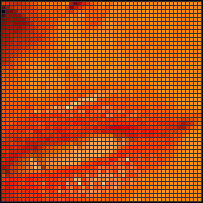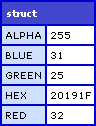# Getting RGBA Pixel Data From ColdFusion Images Using GetPixels()

By on
Tags:

This weekend, I added two more user defined functions to the ImageUtils.cfc ColdFusion component for image manipulation:

• GetPixel()
• GetPixels()

GetPixels() takes a ColdFusion image and returns an array or arrays that contains a structure for each sampled pixel. These structures contain a Red, Green, Blue, Alpha, and Hex key for the Red, Green, Blue, and Alpha channels respectively and the Hex for the 6 digit hexadecimal encoding of the color. By default, if you just pass in the ColdFusion image, GetPixels() will return the pixel data for the entire image. However, you have the option to pass in a starting X and Y coordinate as well as the width and height of the desired sample area:

GetPixels( Image [, X [, Y [, Width [, Height ] ] ] ] ) :: Array

This leverages the underlying Java AWT buffered image object; I am no master of this library, so this might not be the most performant utility function. It will, however, provide me with tools that I will leverage in the authoring of future utility functions.

Let's take a look at an example of how this works. In the demo below, we will take this image:... and sample a 50px by 50px area in the middle of the image. Then, we will loop over that sample and output it in 3 by 3 divs:

``````<!DOCTYPE html PUBLIC "-//W3C//DTD XHTML 1.0 Transitional//EN" "http://www.w3.org/TR/xhtml1/DTD/xhtml1-transitional.dtd">
<html>
<title>GetPixels()</title>

<style type="text/css">

div.canvas {
background-color: #333333 ;
border: 1px solid #000000 ;
float: left ;
padding: 1px 0px 0px 1px ;
}

div.row {
clear: both ;
height: 3px ;
margin-bottom: 1px ;
overflow: hidden ;
}

div.pixel {
float: left ;
font-size: 1px ;
height: 3px ;
line-height: 1px ;
margin: 0px 1px 0px 0px ;
overflow: hidden ;
width: 3px ;
}

</style>
<body>

<!--- Create the image utils object. --->
<cfset objImageUtils = CreateObject( "component", "imageutilsroot.imageUtils" ).Init() />

<!--- Read in the target image. --->
<cfimage
source="../subject.jpg"
name="objImage"
/>

<!--- Read in 50 x 50 pixels. --->
<cfset arrPixels = objImageUtils.GetPixels(
objImage,
200,
185,
50,
50
) />

<cfoutput>

<div class="canvas">

<!--- Loop or y axis. --->
<cfloop
index="intY"
from="1"
to="#ArrayLen( arrPixels )#"
step="1">

<div class="row">

<!--- Loop over x axis. --->
<cfloop
index="intX"
from="1"
to="#ArrayLen( arrPixels[ intY ] )#"
step="1">

<!--- Get the pixel short-hand from the array. --->
<cfset objPixel = arrPixels[ intY ][ intX ] />

<div
class="pixel"
style="background-color: ###objPixel.Hex#;"
></div>

</cfloop>

</div>

</cfloop>

</div>

</cfoutput>

</body>
</html>
``````

Running this code, we get the following pixilated output:As you can see, each pixel is drawn using a 3px by 3px div with an explicit background color using the HEX value returned from the function.

Here is the code behind this functionality:

``````<cffunction
name="GetPixels"
access="public"
returntype="array"
output="false"
hint="Returns a two dimensional array of RGBA values for the image. Array will be in the form of Pixels[ Y ][ X ] where Y is along the height axis and X is along the width axis.">

<!--- Define arguments. --->
<cfargument
name="Image"
type="any"
required="true"
hint="The ColdFusion image object whose pixel map we are returning."
/>

<cfargument
name="X"
type="numeric"
required="false"
default="1"
hint="The default X point where we will start getting our pixels (will be translated to 0-based system for Java interaction)."
/>

<cfargument
name="Y"
type="numeric"
required="false"
default="1"
hint="The default Ypoint where we will start getting our pixels (will be translated to 0-based system for Java interaction)."
/>

<cfargument
name="Width"
type="numeric"
required="false"
default="#ImageGetWidth( ARGUMENTS.Image )#"
hint="The width of the area from which we will be sampling pixels."
/>

<cfargument
name="Height"
type="numeric"
required="false"
default="#ImageGetHeight( ARGUMENTS.Image )#"
hint="The height of the area from which we will be sampling pixels."
/>

<!--- Define the local scope. --->
<cfset var LOCAL = {} />

<!--- Define the pixels array. --->
<cfset LOCAL.Pixels = [] />

<!---
Get the image data raster. This holds all the data
individual pixel data.
--->
<cfset LOCAL.Raster = ImageGetBufferedImage( ARGUMENTS.Image ).GetRaster() />

<!---
Now, starting at our Y position, we want to move down
each column (Y-direction) and gather the pixel values
for each row along the Y-axis.
--->
<cfloop
index="LOCAL.Y"
from="#ARGUMENTS.Y#"
to="#(ARGUMENTS.Y + ARGUMENTS.Height - 1)#"
step="1">

<!---
Create a nested array for this row of pixels.
Remember, the data will be in the Pixel[ Y ][ X ]
array format.
--->
<cfset ArrayAppend(
LOCAL.Pixels,
ArrayNew( 1 )
) />

<!---
Loop over this row of pixels at this given Y-axis
position.
--->
<cfloop
index="LOCAL.X"
from="#ARGUMENTS.X#"
to="#(ARGUMENTS.X + ARGUMENTS.Width - 1)#"
step="1">

<!---
Create an In-array to be used to retreive the
color data. Each element will be used for the
Red, Green, Blue, and Alpha channels respectively.
Be sure to use the JavaCast() here rather than
when we pass in the array to the GetPixel()
method. This way, we don't lose the reference
to the array value.
--->
<cfset LOCAL.PixelArray = JavaCast(
"int[]",
ListToArray( "0,0,0,0" )
) />

<!--- Get the pixel data array. --->
<cfset LOCAL.Raster.GetPixel(
JavaCast( "int", (LOCAL.X - 1) ),
JavaCast( "int", (LOCAL.Y - 1) ),
LOCAL.PixelArray
) />

<!---
Now that we have an index-based pixel data
array, let's convert the the data into a keyed
struct that will be more user friendly.
--->
<cfset LOCAL.Pixel = {
Red = LOCAL.PixelArray[ 1 ],
Green = LOCAL.PixelArray[ 2 ],
Blue = LOCAL.PixelArray[ 3 ],
Alpha = LOCAL.PixelArray[ 4 ],
Hex = ""
} />

<!--- Set the HEX value based on the RGB. --->
<cfset LOCAL.Pixel.Hex = UCase(
Right( "0#FormatBaseN( LOCAL.Pixel.Red, '16' )#", 2 ) &
Right( "0#FormatBaseN( LOCAL.Pixel.Green, '16' )#", 2 ) &
Right( "0#FormatBaseN( LOCAL.Pixel.Blue, '16' )#", 2 )
) />

<!--- Add this pixel data to curren row. --->
<cfset ArrayAppend(
LOCAL.Pixels[ ArrayLen( LOCAL.Pixels ) ],
LOCAL.Pixel
) />

</cfloop>

</cfloop>

<!--- Return pixels. --->
<cfreturn LOCAL.Pixels />
</cffunction>
``````

In addition to this function, I also created a GetPixel() function that utilizes the GetPixels() function to return the RGBA values for a single pixel:

``````<cffunction
name="GetPixel"
access="public"
returntype="struct"
output="false"
hint="Returns a struct containing the given pixel RGBA data.">

<!--- Define arguments. --->
<cfargument
name="Image"
type="any"
required="true"
hint="The ColdFusion image object whose pixel data map we are returning."
/>

<cfargument
name="X"
type="numeric"
required="true"
hint="The X coordinate of the pixel that we are returning."
/>

<cfargument
name="Y"
type="numeric"
required="true"
hint="The Y coordinate of the pixel that we are returning."
/>

<!--- Define the local scope. --->
<cfset var LOCAL = {} />

<!--- Get the pixels array for a 1x1 area. --->
<cfset LOCAL.Pixels = GetPixels(
ARGUMENTS.Image,
ARGUMENTS.X,
ARGUMENTS.Y,
1,
1
) />

<!--- Return the first returned pixel. --->
<cfreturn LOCAL.Pixels[ 1 ][ 1 ] />
</cffunction>
``````

This returns a single instance of the pixel structure which looks like this:Want to use code from this post? Check out the license.That is really cool! (though I can't quite think of a use for it)@Steve,

I actually started coding it with another method in mind. Soon, I will come out with TrimCanvas(). This will remove rows and columns from the edges until it hits "real image content". Basically, this is a way of shrinking a canvas to the smallest possible box without cropped image data.

In FireWorks, this would be CTRL+ALT+T. I figure, I can perform this by sampling pixels until I find one that is not the same as the background color.

Now, not sure if that function will have a use, but I think it will, especially when you do a lot of programmatic pasting of images.*that* is nice! (definitely useful)

So you can get the background color of an image separately?

Can you change the background color for index transparency gifs?@Steve,

I am not sure if you can get the background color of an image since I don't think that is really stored in any capacity once the background is drawn. I might have to end up passing in the background color as a hint:

TrimCanvas( Image, CanvasColor )

As far as working with transparent GIF images, I guess you could create an alpha canvas and then paste the GIF onto that canvas? I am not exactly sure what you are asking.Sorry, my thought only made sense on the assumption that you could have read and write access to a background color property which doesn't seem to be the case.

Still, very nifty. I am eager to see how it comes out.Sweet hack.Just posted a Tweet asking for help getting the values of pixels from an image.

Knew i should have just looked on here first.

Excellent post.. and exactly what i was looking for@Jbuda,Hi Ben

Just looking at the code.. how would i get the dominant colour on the entire image?

Would i just slice the image up and the loop through each fragment and tally up the colours in an struct?

The struct with most colours is the winner?@Jbuda,

I am not sure what you mean by dominant color? You mean like between Red, Green, and Blue? Or are you talking about a particular RGB combination?Thanks Ben for this article! GetPixels() helped me to print graphics on a Plastic ID Card Printer. I needed each pixel of the graphic to generate the appropriate hexa code for the printer. The other article about InputBaseN and FormatBaseN was helpful too!@Tony,

Awesome my man - glad to help.Has this been testing with CF9? No matter what I use for source images I get:

Coordinate out of bounds!

Line 1225.@Raymond,

Hmm, I have not tried this in CF9 yet. I'm having a little trouble getting my CF9 instance to run with certain tags.